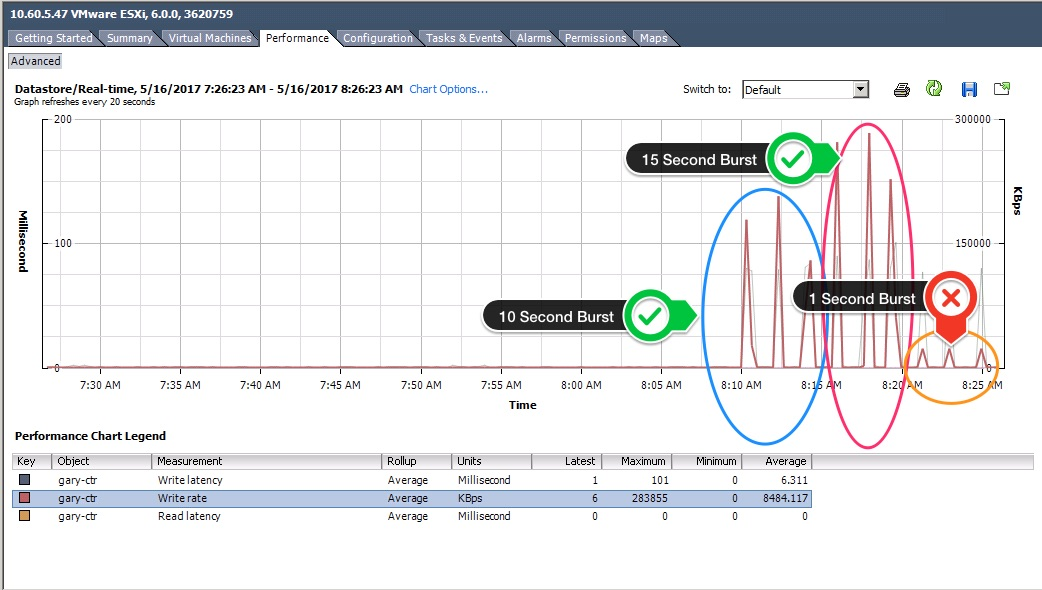High Response time and low throughput in vCenter performance charts.Often we are presented with a vCenter screenshot, and an observation that there are “high latency spikes”.  In the example, the response time is indeed quite high – around 80ms.

Why is that?  In this case – there are bursts of large IO’s with high queue depths.  32 Outstanding IO’s and each IO is 1MB. For such a workload 80ms is OK.  By comparison for a 8K write with 1 outstanding IO, the response time would be closer to .8ms.  Anyhow, the workload here has a response time of 80ms,  however the throughput from the application (in this case fio) is a reasonable 400MB/s.

The problem is that 400MB/s is  not what vCenter reports.  Depending on the burst duration vCenter can vastly under-report the actual throughput.  In the worse case, vCenter reports the 80ms latency – for only ~17.5MB/s.  Littles law tells us that the expected throughput is (1/.08)*32 IOPS and since each IOP is 1MB in size – we should see roughly 400MB/s.

It turns out that vCenter is averaging the throughput over the sample time of 20 seconds – but the response time (since it is not a rate) is averaged over the number of IOPS in the time period – not the time period itself.   So, where the burst is less than 20 seconds, throughput is inaccurate (but response time is accurate).  This is not a criticism of vCenter – pretty much all monitoring software does this (including iostat at 1second granularity – where IO bursts are less than one second).

Output from fio our “Application”.

Our “application” which is fio, accurately records the achieved throughput and response times.

1 Second Burst
wr3: (g=0): rw=write, bs=1M-1M/1M-1M/1M-1M, ioengine=libaio, iodepth=32
fio-2.1.3
Starting 1 process

wr3: (groupid=0, jobs=1): err= 0: pid=5046: Tue May 16 15:54:23 2017
write: io=436224KB, bw=404285KB/s, iops=394, runt= 1079msec
slat (usec): min=58, max=180, avg=129.55, stdev=18.61
clat (msec): min=10, max=151, avg=80.58, stdev=16.41
lat (msec): min=10, max=151, avg=80.71, stdev=16.41
10 Second Burst
wr3: (g=0): rw=write, bs=1M-1M/1M-1M/1M-1M, ioengine=libaio, iodepth=32
fio-2.1.3
Starting 1 process

wr3: (groupid=0, jobs=1): err= 0: pid=5029: Tue May 16 15:54:00 2017
write: io=4005.0MB, bw=407019KB/s, iops=397, runt= 10076msec
slat (usec): min=57, max=242, avg=132.72, stdev=19.73
clat (msec): min=17, max=161, avg=80.34, stdev= 7.05
lat (msec): min=17, max=161, avg=80.47, stdev= 7.05